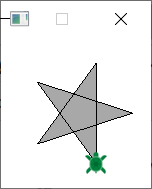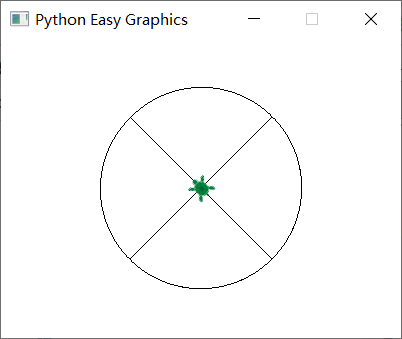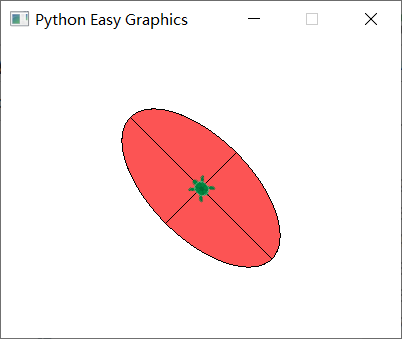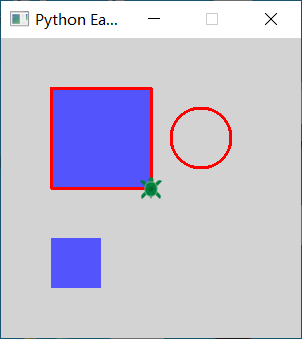## Fill the drawings¶

Drawings between begin_fill() and end_fill() will be filled using the fill color. Unclosed shapes will get closed automatically.

By default the fill color is light gray. You can use easygraphics set_fill_color() to change it.

The following program draw and fill a star. Note that we use the FillRule.WINDING_FILL rule to fill the whole star.

```from easygraphics.turtle import *
from easygraphics import *

def main():
create_world(150, 150)

setxy(20,-50)
set_fill_rule(FillRule.WINDING_FILL)
begin_fill()
for i in range(5):
fd(100)
lt(144)
end_fill()

pause()
close_world()

easy_run(main)
```## Move in arcs¶

move_arc(radius,angle) moves the turtle in a arc path.

The center is radius units left of the turtle. That is, if radius > 0, the center is on the left of the turtle; if radius < 0, the center is on the right of the turtle.

Angle is the value of the angle at the circle’s center. If angle > 0, the turtle moves forward around the center; if angle < 0, the turtle moves backward around the center. So:

• if angle > 0 and radius > 0, the turtle moves forward and turns counter-clockwise;
• if angle > 0 and raidus < 0, the turtle move forward and turns clockwise;
• if angle <0 and radius > 0, the turtle moves backward and turns clockwise;
• if angle <0 and radius < 0, the turtle moves backward and turns counter-clockwise.
```from easygraphics.turtle import *

def main():
create_world(400, 300)
set_speed(10)

lt(45)

fd(100)
lt(90)
move_arc(100, 90)
lt(90)
fd(100)
lt(90)

fd(100)
rt(90)
move_arc(-100, 90)
rt(90)
fd(100)
rt(90)

bk(100)
rt(90)
move_arc(100, -90)
rt(90)
bk(100)
rt(90)

bk(100)
lt(90)
move_arc(-100, -90)
lt(90)
bk(100)
lt(90)

pause()

close_world()

easy_run(main)
```## Move in ellipse arcs¶

“radius_left” is the radius of the ellipse on the direction perpendicular to the turtle’s orientation, it can be postive or negtive;”radius_top” is the radius of the ellipse on the direction parallel to the turtle’s orientation, it must be postive.

The center is radius_left units left of the turtle. That is, if radius_left > 0, the center is on the left of the turtle; if radius_left < 0, the center is on the right of the turtle.

If angle > 0, the turtle moves forward around the center; if angle < 0, the turtle moves backward around the center. So:

• if angle > 0 and radius_left > 0, the turtle moves forward and turns counter-clockwise;
• if angle > 0 and radius_left < 0, the turtle move forward and turns clockwise;
• if angle <0 and radius_left > 0, the turtle moves backward and turns clockwise;
• if angle <0 and radius_left < 0, the turtle moves backward and turns counter-clockwise.
```from easygraphics.turtle import *
from easygraphics import *

def main():
create_world(400, 300)
set_speed(5)

lt(45)

set_fill_color(Color.LIGHT_RED)
begin_fill()
fd(100)
lt(90)
move_ellipse(100, 50, 90)
lt(90)
fd(50)
lt(90)
end_fill()

begin_fill()
fd(100)
rt(90)
move_ellipse(-100, 50, 90)
rt(90)
fd(50)
rt(90)
end_fill()

begin_fill()
bk(100)
rt(90)
move_ellipse(100, 50, -90)
rt(90)
bk(50)
rt(90)
end_fill()

begin_fill()
bk(100)
lt(90)
move_ellipse(-100, 50, -90)
lt(90)
bk(50)
lt(90)
end_fill()

pause()

close_world()

easy_run(main)
```## Use easygraphics functions¶

Most of the easygraphics functions can be used in turtle graphics.

The following program use easygraphics functions to set the line width and colors, draw a circle, and fill a rectangle.

```from easygraphics.turtle import *
from easygraphics import *

def main():
create_world(300,300)

set_line_width(3)
set_color("red")
set_background_color("lightgray")
set_fill_color(Color.LIGHT_BLUE)

begin_fill()
for i in range(4):
fd(100)
lt(90)
end_fill()

circle(50,50,30)
fill_rect(-100,-100,-50,-50)
pause()
close_world()

easy_run(main)
```